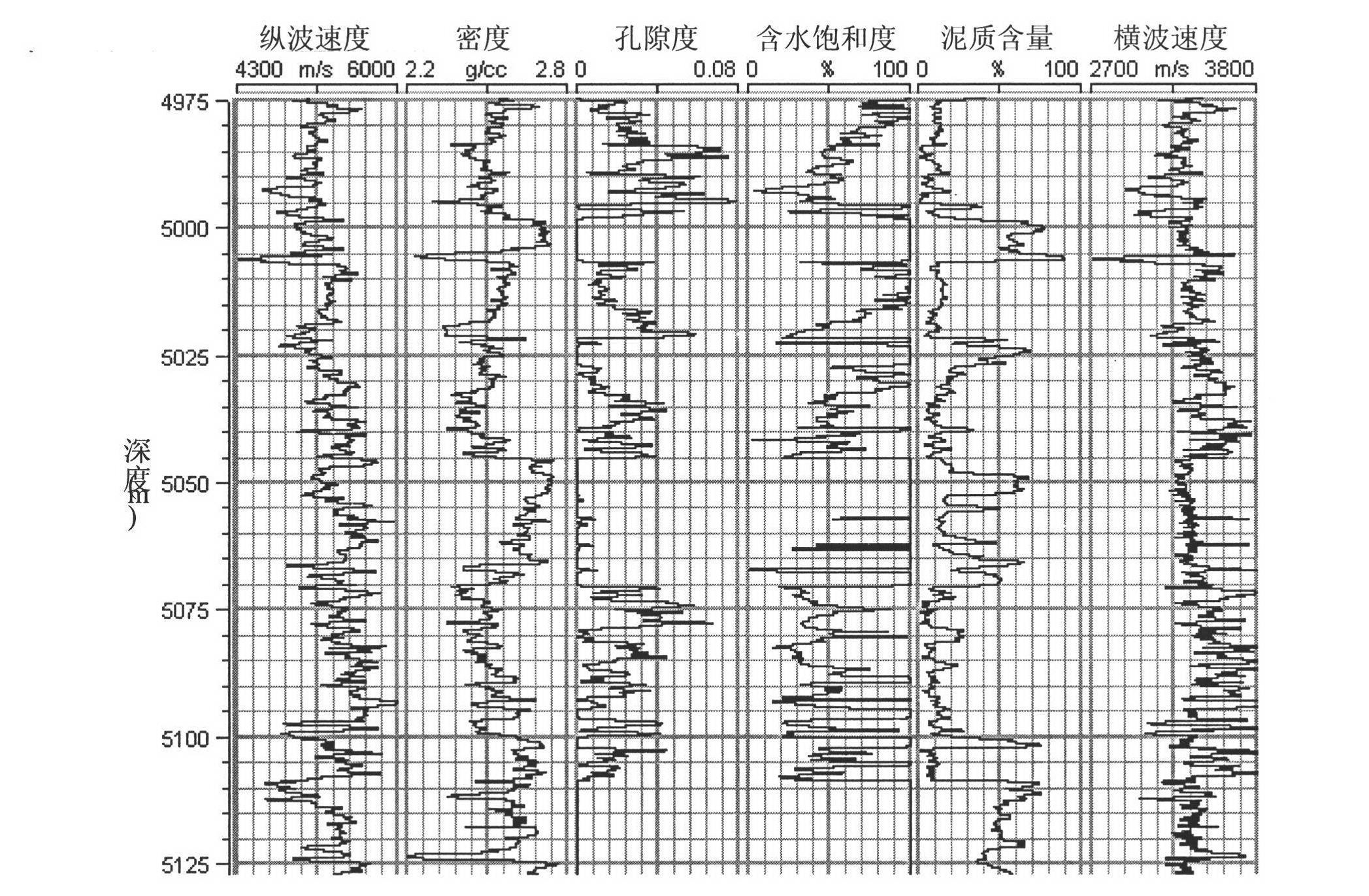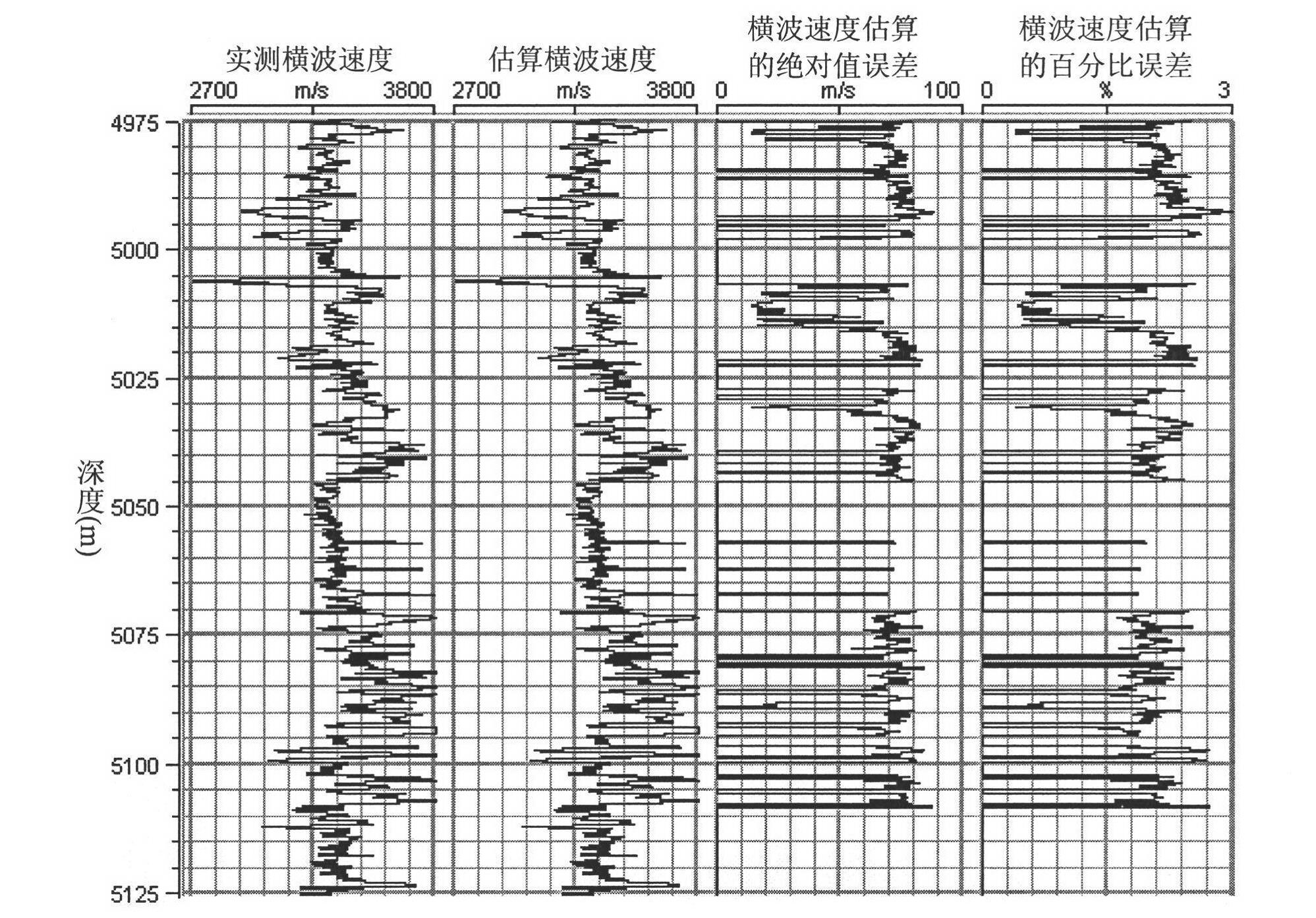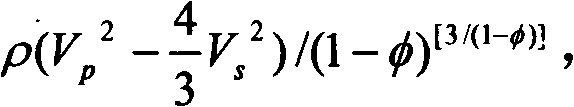# Method for estimating velocity of transverse waves based on inversion of equivalent elastic modulus for self-adapting matrix minerals

## A technology of shear wave velocity and self-adaptation, which is applied in the fields of seismology and seismic signal processing for well logging records. It can solve the problems of large elastic modulus value variation range, high cost, and difficult setting, and achieve high calculation accuracy. , reduce multi-solution, and reduce the number of effects

Inactive Publication Date: 2012-02-15
CHENGDU UNIVERSITY OF TECHNOLOGY
1 Cites 39 Cited by

## AI-Extracted Technical Summary

### Problems solved by technology

Conventional methods for setting the elastic modulus of matrix minerals mainly fall into the following five categories: (1) For single-matrix mineral rocks, refer to domestic and foreign petrophysical test values ​​for setting, and the defect is that the elastic modulus value of the same matrix mineral (2) For multi-matrix mineral rocks, use equivalent medium theory (such as V-R-H average) to calculate the equivalent elastic modulus (comprehensive influence value of various matrix minerals), and its defects It is difficult to accurately set the percentage content and elastic modulus value of each matrix mineral; (3) Based on the logging data, the statistical method is used to select the depth section with rela...
View more

## Abstract

The invention discloses a method for estimating the velocity of transverse waves based on the inversion of an equivalent elastic modulus for self-adapting matrix minerals, which belongs to the field of exploration geophysics, and used for solving the problem that in a conventional method for estimating the velocity of transverse waves based on a Xu-White model, an elastic modulus for matrix minerals is difficult to set accurately. The method comprises the following steps of: (1) setting the Poisson ratio of a dry core sample at a single logging depth point and the value selection range and initial value of an equivalent bulk modulus for matrix minerals; (2) carrying out inversion solution on a same fluid item from two different angles by using two different fluid item calculation methods so as to invert an optimal Poisson ratio of the dry core sample and an optimal equivalent bulk modulus and an optimal equivalent shear modulus for matrix minerals; and (3) estimating the velocity of transverse waves by using a simplified Xu-White model. In the method disclosed by the invention, the equivalent elastic modulus for matrix minerals is taken as an object of study, through reducing the number of target parameters, the precision and reliability of the method for estimating the velocity of transverse waves are effectively improved.

Application Domain

Seismic signal processingSeismology for water-logging

Technology Topic

Bulk modulusCore sample +12

## Image

•••## Examples

• Experimental program(1)

### Example Embodiment

 The shear wave velocity estimation method based on the inversion of the equivalent elastic modulus of adaptive matrix minerals, the specific implementation steps are as follows:
 (1) Taking a single logging depth point as the research object, according to the logging data (P-wave velocity, density and porosity) at that depth point, the empirical relationship between the longitudinal wave velocity and the shear wave velocity of sand and mudstone is used (Castagna, 1993) to estimate Initial shear wave velocity, and calculate the range, initial value and increment of the Poisson’s ratio of the dry rock and the equivalent bulk modulus of the matrix mineral at the logging depth point.
 The empirical relationship between longitudinal wave velocity and shear wave velocity of sand and mudstone (Castagna, 1993) is shown in formula 1.
 V s = 0.804V p -0.856 (1)
 Where V p Represents longitudinal wave velocity, V s Represents transverse wave velocity.
 Poisson's ratio of dry rock (σ dry The value range of) is set to 0.0~0.4 (the value range of Poisson’s ratio of common sedimentary rocks). The initial value is generally equal to the lower or upper limit of the value range, and the increment is generally between 0.01 and 0.05. between.
 The calculation formula for the lower limit and upper limit of the equivalent bulk modulus range of the matrix mineral is shown in formula 2.
 lower limit: ρ ( V p 2 - 4 3 V s 2 ) / ( 1 - φ ) [ 3 / ( 1 - φ ) ] , Upper limit: [ ρ ( V p 2 - 4 3 V s 2 ) + aφ ] / ( 1 - φ ) [ 3 / ( 1 - φ ) ] - - - ( 2 ) Where a φ Is a correction item related to porosity, a is a constant (generally between 100 and 400), ρ and φ are the density and porosity obtained by logging. The initial value is generally equal to the lower or upper limit of the value range. The increment is generally between 0.0 and 1.0 GPa.
 (2) Two different fluid term calculation methods (based on the Gassmann equation and Gassmann-Boit-Geertsman equation and the method based on the fluid identification factor proposed by Russell et al. in 2003) are used to calculate the fluid term at the same time, and set the above The absolute value of the difference between the two fluid terms is the inversion objective function, and the method of finding the global optimal solution is used to invert the optimal Poisson’s ratio of dry rock and the equivalent bulk modulus of the matrix mineral, when the value of the objective function < Set the threshold (selected according to the calculation accuracy and calculation efficiency, the value is generally between 0 and 0.5), the calculation is stopped, and the equivalent bulk modulus of the rock matrix mineral and the Poisson of dry rock at the current depth point are output ratio.
 Calculation method of fluid term based on Gassmann equation and Gassmann-Boit-Geertsman equation:
 The fluid term referred to in the present invention is the fluid term constituting the longitudinal wave velocity, that is, f in formula 3. Formula 4 is the calculation formula of the fluid term based on Gassmann equation, and Formula 5 is Gassmann-Boit-Geertsman equation, which is a standard one-dimensional quadratic equation with β as a variable. If ρ, V are known p , Σ dry , K 0 , K fl , Φ, β can be solved; then, according to formula 4, the fluid term (f) can be calculated.
 V p = S + f ρ , S = K dry + 4 3 u dry = λ dry + 2 u dry - - - ( 3 )
 f = β 2 M = ( 1 - K dry K 0 ) 2 φ K fl + 1 - φ K 0 - K dry K 0 2 , β = 1 - K dry K 0 , 1 M = β - φ K 0 + φ K fl - - - ( 4 )
 ( Y - 1 ) β 2 + [ Yφ ( K 0 K fl - 1 ) - Y + N K 0 ] β - φ ( Y - N K 0 ) ( K 0 K fl - 1 ) = 0 , Y = 3 ( 1 - σ dry ) ( 1 + σ dry ) , N = ρV P 2 - - - ( 5 )
 In formula 3, formula 4 and formula 5, S represents the framework term, f represents the fluid term, ρ represents the density of fluid-saturated rock (take the logging density value), and K dy , U dry , Λ dry And σ dry Respectively represent the bulk modulus, shear modulus, Lame constant and Poisson’s ratio of dry rock, K 0 Represents the equivalent bulk modulus of the matrix mineral, K fl Represents the bulk modulus of the fluid, and φ represents the porosity.
 The calculation method of fluid term based on the fluid identification factor derived by Russell et al. in 2003:
 ρf = ( Z p 2 - c Z s 2 ) , c = ( V p V s ) dry 2 = 2 ( 1 - σ dry ) 1 - 2 σ dry , Z p =ρV p ,Z s =ρV s (6)
 Ρf in formula 6 is the fluid identification factor, ρ, Z p And Z s They represent the density, P-wave impedance and S-wave impedance of fluid-saturated rocks. If V is known p , V s , Ρ and σ dry , The fluid term (f) can be calculated.
 (3) Based on the Poisson's ratio of the dry rock and the equivalent bulk modulus of the matrix mineral obtained in step 2 inversion, the linear average formula (Equation 7) and Gassmann equation (Equation 8) based on the critical porosity model of Nur (1995) )Calculate the equivalent shear modulus (μ 0 ).
 μ 0 = μ dry ( 1 - φ φ c ) , μ dry = 4 3 K dry ( S - 1 ) , S = 3 ( 1 - σ dry ) 1 + σ dry - - - ( 7 )
 K dry = K sat ( φK 0 K fl + 1 - φ ) - K 0 φK 0 K fl + K sat K 0 - 1 - φ , K sat = ρ ( V p 2 - 4 3 V s 2 ) - - - ( 8 )
 In Equation 7, μ dry And σ dry Represents the shear modulus and Poisson's ratio of dry rock, φ c Represents critical porosity (generally taken as 0.40).
 (4) Based on the equivalent bulk modulus and equivalent shear modulus of the matrix minerals obtained in steps 2 and 3, the simplified Xu-White model (Keys and Xu, 2002) is used to estimate the shear wave velocity. The calculation formula is shown in Equation 9 And formula 10.
 V s = μ d / ρ , μ d =μ 0 (1-φ) q , q = 1 5 X l = s , sh υ l F iijj ( α l ) - - - ( 9 )
 F iijj ( α ) = 2 F 3 + 1 F 4 + F 4 F 5 + F 6 F 7 - F 8 F 9 F 2 F 4 - - - ( 10 - 1 )
 F 2 = 1 + A [ 1 + 3 2 ( g + γ ) - R 2 ( 3 g + 5 γ ) ] + B ( 3 - 4 R ) + A 2 ( A + 3 B ) ( 3 - 4 R ) [ g + γ - R ( g - γ + 2 υ 2 ) ] - - - ( 10 - 2 )
 F 3 = 1 + A 2 [ R ( 2 - γ ) - 1 + α 2 α 2 g ( R - 1 ) ] - - - ( 10 - 3 )
 F 4 = 1 + A 4 [ 3 γ + g - R ( g - γ ) ] - - - ( 10 - 4 )
 F 5 = A [ R ( g + γ - 4 3 ) - g ] + Bγ ( 3 - 4 R ) - - - ( 10 - 5 )
 P 6 =1+A[1+g-R(γ+g)]+B(1-γ)(3-4R) (10-6)
 F 7 = 2 + A 4 [ 9 γ + 3 g - R ( 5 γ + 3 g ) ] + Bγ ( 3 - 4 R ) - - - ( 10 - 7 )
 F 8 = A [ 1 - 2 R + g 2 ( R - 1 ) + υ 2 ( 5 R - 3 ) ] + B ( 1 - γ ) ( 3 - 4 R ) - - - ( 10 - 8 )
 F 9 =A[g(R-1)-Rγ]+Bγ(3-4R) (10-9)
 A=-1, B=0, R = 3 μ 0 3 K 0 + 4 μ 0 , g = α 2 1 - α 2 ( 3 γ - 2 ) , γ = α ( 1 - α 2 ) 3 / 2 [ cos - 1 ( α ) - α 1 - α 2 ] - - - ( 10 - 10 )
 In formula 9 and formula 10, υ l And α l Respectively represent the volume percentage of sandstone and mudstone in the rock matrix (calculated based on the logging mud content) and pore flatness (the flatness of sandstone pores is 0.10, and the flattening of mudstone pores is 0.04, which is similar to the conventional Xu-White model The values ​​are the same), F iiij (α l ) Represents the pore flatness α l Eshelby tensor at time.
 (5) Select the next logging depth point and repeat the calculation in steps 1 to 5; stop the calculation until all the logging depth points in the target depth section are calculated.
 (6) Output the shear wave velocity value estimated at each logging depth point in the target depth section.
 figure 1 with figure 2 It is a calculation example of shear wave velocity estimation based on actual logging data. based on figure 1 The five input parameters shown, including longitudinal wave velocity, density, porosity, water saturation, and shale content, are calculated in strict accordance with the 6 steps of the specific embodiment of the present invention to obtain figure 2 The estimated shear wave velocity curve in, then use the measured shear wave velocity and the estimated shear wave velocity to calculate the absolute value of the error (|estimated shear wave velocity-measured shear wave velocity|) and error percentage The values ​​of the parameters involved in the above calculation are as follows:
 ①The value of the constant a for calculating the equivalent bulk modulus of the matrix mineral: 200;
 ②Increase of equivalent bulk modulus of matrix mineral: 1.0GPa;
 ③The value range of Poisson's ratio of dry rock: 0.0~0.4;
 ④Increase of Poisson's ratio of dry rock: 0.02;
 ⑤ Threshold: 0.5;
 ⑥Sandstone flatness: 0.10, mudstone flatness: 0.04; critical porosity: 0.40.

## PUM## Description & Claims & Application Information

We can also present the details of the Description, Claims and Application information to help users get a comprehensive understanding of the technical details of the patent, such as background art, summary of invention, brief description of drawings, description of embodiments, and other original content. On the other hand, users can also determine the specific scope of protection of the technology through the list of claims; as well as understand the changes in the life cycle of the technology with the presentation of the patent timeline. Login to view more.

## A compliant track suspension system

ActiveCN109094674Alow costreduce the number
Owner:BEIJING LESEN TECH CO LTD

## Single impeller twin-stage blwor

InactiveCN107795497Areduce the numberreduce cumulative error
Owner:福安市康盛电机有限公司

Owner:KMW INC

## Fixing device and image forming apparatus

ActiveCN103309216Areduce the number

Owner:刘小祥

## Classification and recommendation of technical efficacy words

• reduce the number
• reduce ambiguity

## Passive network performance measuring system and page identification method thereof

ActiveCN102361484Areduce the numberlow cost
Owner:SHANGHAI JIAO TONG UNIV

## Method and system for detecting single-plate on position

ActiveCN1912641Areduce the numberReliable presence detection
Owner:HUAWEI TECH CO LTD

## Multiband matching circuit, radio frequency circuit, antenna system and mobile terminal

InactiveCN106571788AReduce the difficulty of debuggingreduce the number
Owner:QINGDAO HISENSE MOBILE COMM TECH CO LTD

Owner:SIEMENS AG

## Character selection method based on particle swarm optimization algorithm

Owner:NANJING UNIV OF POSTS & TELECOMM
Who we serve
• R&D Engineer
• R&D Manager
• IP Professional
Why Eureka